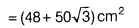# A field is in the shape of given figure, in which ABCD is a rectangle and the triangles

A field is in the shape of given figure, in which ABCD is a rectangle and the triangles APB, BCQ, CDR and ADS, whose make out side of rectangle are equilateral. Find the area of field.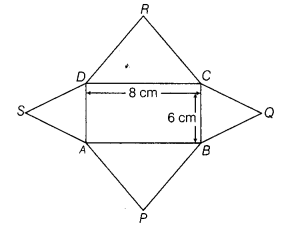From the given figure.
sides of the rectangle are 8 cm and 6 cm.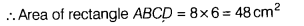[∵ area of rectangle = length x breadth]
Side of triangles APB and CDR is 8 cm.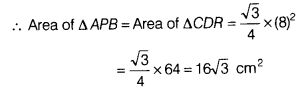Side of triangles BCQ and ADS is 6 cm.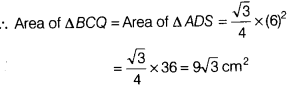Now, area of field=Area of rectangle ABCD+ Area of ∆ APB + Area of ∆BCQ + Area of ∆CDR + Area of ∆ ADS
= 48+16√3 +9√3 +16√3+9√3